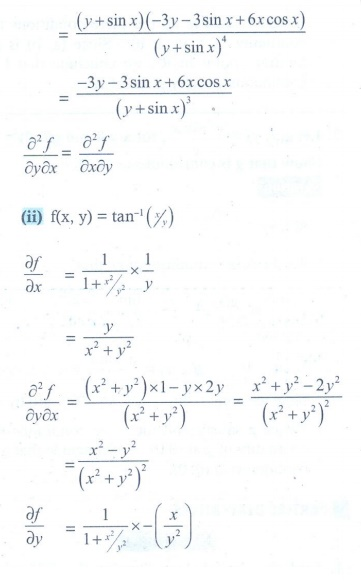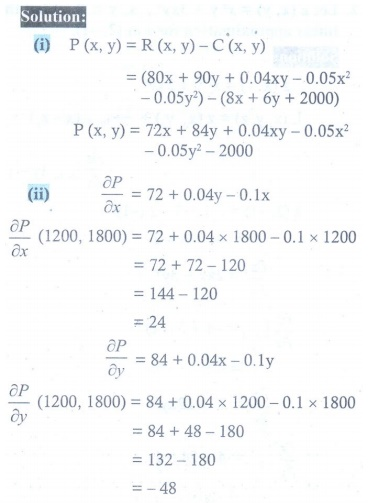Home | | Maths 12th Std | Exercise 8.4: Partial Derivatives

# Exercise 8.4: Partial Derivatives

Maths Book back answers and solution for Exercise questions - Mathematics : Partial Derivatives : Exercise Problem Questions with Answer, Solution

EXERCISE 8.4

1. Find the partial derivatives of the following functions at the indicated points.

(i) f (x, y ) = 3x2 ŌłÆ 2xy + y2 + 5x + 2,       (2, ŌłÆ5)

(ii) gx, y ) = 3x2 + y2 + 5x + 2,         (1, ŌłÆ2)

(iii) h(x,y,z) = x sin(xy) + z2x       ( 2,ŽĆ/4,1)

(iv) G ( x, y ) = ex+3y log(x2 + y2 ), (ŌłÆ1,1)2. For each of the following functions find the fx fy , and show that fxy = fyx.

(i) f (x , y) = 3x / y+sinx

(ii) f (x , y) = tanŌłÆ1 (x/ y)

(iii) f (x, y ) = cos(x2 ŌłÆ 3xy)3.4. If U ( x, y , z) = log(x3 + y3 + z3 ) , find ŌłéU/Ōłéx , ŌłéU/Ōłéy , and ŌłéU/Ōłéz .5. For each of the following functions find the gxy , gxx , gyy and gyx.

(i) g ( x, y ) = xey + 3x2y

(ii) g x, y ) = log(5x + 3y)

(iii) g ( x, y ) = x2 + 3xy ŌłÆ 7+ cos(5x)6. Let w( x, y , z) = 1 / ŌłÜ[x2 + y2 + z2] , (x , y, z) ŌēĀ (0, 0, 0) . Show that7. If V ( x, y ) = ex(x cos y ŌłÆ y sin y) , then prove that Ōłé2V/Ōłéx2 + Ōłé2V/Ōłéy2= 0.8. If w( x, y ) = xy + sin( xy) , then prove that9. If v x, y , z) = x3 + y3 + z3 + 3xyz , show that Ōłé2v / ŌłéyŌłéz  = Ōłé2v / ŌłézŌłéy.10. A firm produces two types of calculators each week, x number of type A and y number of type . The weekly revenue and cost functions (in rupees) are ŌäØ ( x, y ) = 80+ 90 y + 0.04xy ŌłÆ 0.05x2 ŌłÆ 0.05 y2 and C ( x, y ) = 8+ 6 y + 2000 respectively.

(i) Find the profit function P ( xy) ,

(ii) Find ŌłéP/Ōłéx (1200,1800) and Ōłép/Ōłéy (1200,1800) and interpret these results.